Home > CCA2 > Chapter 8 > Lesson 8.2.3 > Problem8-104

8-104.
1.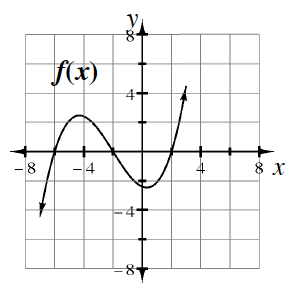In parts (a) through (d) below, for each polynomial function f(x), the graph of f(x) is shown. Based on this information, state the number of linear and quadratic factors the factored form of its equation should have and how many real and complex (non-real) solutions f(x) = 0 might have. (Assume a polynomial function of the lowest possible degree for each one.)

2. Example: f(x) at right will have three linear factors, therefore three real roots and no complex roots. Homework Help ✎

 a.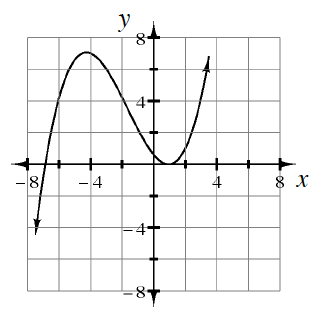b.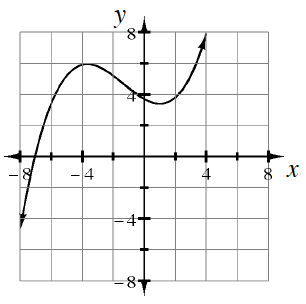c.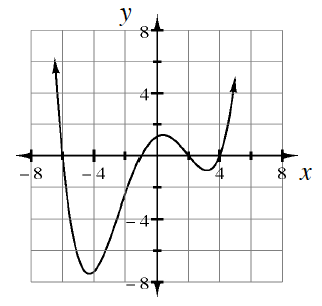d.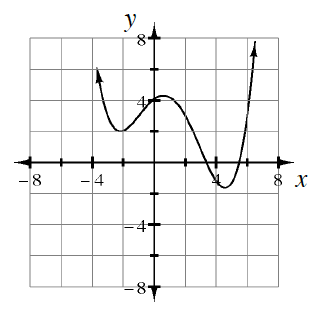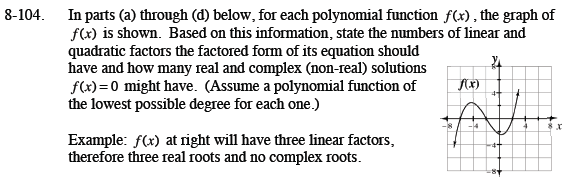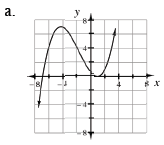There will be three linear factors (one repeated), therefore two real (one single, one double) and zero complex (non-real) roots.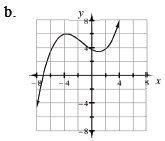There will be one linear factor and one quadratic factor, therefore one real and two complex (non-real) roots.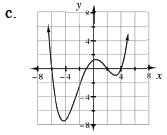There will be four linear factors, therefore four real and zero complex (non-real) roots.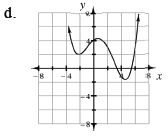There will be two linear and one quadratic factor, therefore two real and two complex (non-real) roots.